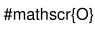# Calligraphic symbols

Dear all,

we’re trying to write a calligraphic R (\cal{R} in LateX) in the title of a histogram. I understand that’s currently not possible, but would be happy to be proven wrong.

I’ve seen old messages requesting this, but haven’t seen the solution. I did find a promise that it might be possible to do this in ROOT 6.0, but we need a solution on the time scale of days, rather than weeks.

If there is no solution to this problem in ROOT, we may simply post-process the PS file. The calligraphic R is actualy not part of the /symbol set either, so if anyone knows how to introduce the symbol in the PS file by hand, that’s very welcome…

Regards, Marcel

Actually it is possible now with the new class TMathText.
Have a look at: \$ROOTSYS/tutorials/graphics/tma*.C

It is in version 5.34… I do not see doc yet on the reference manual online. I will check.

Hi Marcel,

As mentioned by Olivier, this should be possible in TMathText, and using “\mathscr{R}” (just like TeX).

Note that in TeX nomenclature, there is a distinction between calligraphic/\mathcal (brushstroke) and script/\mathscr (metallic quill/English script). Because Unicode has script but no brushstroke code points, only \mathscr is implemented.

Best,

Yue Shi Lai

You will only need to define your histogram title like:

"Histogram title with \\mathscr{R} inside"

Hi,
I’m having problems in PyROOT (ROOT 6.24/06). In a TMultiGraph I write:

mgGraphs[z].GetXaxis().SetTitle("\\mathscr{O}")


And I get:It works for me:

auto l = new TLatex(.5,.5,"Histogram title with \\mathscr{0} inside");
l->Draw();Not sure how to implement that as a TMultiGraph axis label

{
TGraph *g;
Double_t x = {0,1,2,3,4,5,6,7,8,9};
Double_t y = {1,2,3,4,5,5,4,3,2,1};
TMultiGraph *mg = new TMultiGraph();
for (int i=0; i<3; i++) {
g[i] = new TGraph(10, x, y);
g[i]->SetMarkerStyle(20);
g[i]->SetMarkerColor(i+2);
for (int j=0; j<10; j++) y[j] = y[j]-1;
}
mg->Draw("APL");
mg->GetXaxis()->SetTitle("E_{#gamma} (GeV)");
mg->GetYaxis()->SetTitle("Coefficients");

// Change the axis limits
mg->GetXaxis()->SetLimits(1.5,7.5);
mg->SetMinimum(0.);
mg->SetMaximum(10.);
mg->GetXaxis()->SetTitle("Histogram title with \\mathscr{0} inside")
}


BTW I do not understand why you need such fond for “0”. It will appear the same as a normal “0”

That is what I wrote in the first message basically.

mgGraphs[z].GetXaxis().SetTitle("\\mathscr{O}")


It is not a zero, it is a capital o (letter).

{
TGraph *g;
Double_t x = {0,1,2,3,4,5,6,7,8,9};
Double_t y = {1,2,3,4,5,5,4,3,2,1};
TMultiGraph *mg = new TMultiGraph();
for (int i=0; i<3; i++) {
g[i] = new TGraph(10, x, y);
g[i]->SetMarkerStyle(20);
g[i]->SetMarkerColor(i+2);
for (int j=0; j<10; j++) y[j] = y[j]-1;
}
mg->Draw("APL");
mg->GetXaxis()->SetTitle("E_{#gamma} (GeV)");
mg->GetYaxis()->SetTitle("Coefficients");

// Change the axis limits
mg->GetXaxis()->SetLimits(1.5,7.5);
mg->SetMinimum(0.);
mg->SetMaximum(10.);
mg->GetXaxis()->SetTitle("\\mathscr{O}")
}


You:

mg->GetXaxis()->SetTitle("\\mathscr{O}")


Me:

mgGraphs[z].GetXaxis().SetTitle("\\mathscr{O}") # mgGraphs[z] = ROOT.TMultiGraph()


I think there is not relevant difference in the code. Maybe PyROOT does not support this feature?

Does my macro works for you ?

Yes, using my ROOT installation works, but I seems that in Python it does not work.

Ok. That might be a Python issue. That’s a bit surprising to me but why not. May be @etejedor has an idea.

What about if you translate my macro in to Python ? does it fail too ?

import ROOT

x = [0,1,2,3,4,5,6,7,8,9]
y = [1,2,3,4,5,5,4,3,2,1]

N = 3
g = {}
mg = ROOT.TMultiGraph()

for i in range(N):

g[i] = ROOT.TGraph()
for j in range(len(x)):
g[i].SetMarkerStyle(20)
g[i].SetMarkerColor(i+2)
for j in range(len(x)):
y[j] = y[j] -1

mg.Draw("APL")
mg.GetXaxis().SetTitle("E_{#gamma} (GeV)")
mg.GetYaxis().SetTitle("Coefficients")

mg.GetXaxis().SetLimits(1.5,7.5)
mg.SetMinimum(0.)
mg.SetMaximum(10.)
mg.GetXaxis().SetTitle("\\mathscr{O}")


I did something wrong about the graph points, but the important thing, the calligraphic O is there.

No idea why my original code does not work properly.

Me neither. So to is fixed. Fine.

I’m back again @couet . I modified your code to have a TCanvas and save the graph. Look at this:

So the problem is not in the code, it seems that the problem comes when the canvas is printed as a pdf. Because if I save it as png, calligraphic symbol is ok.

Code here:

void TEST(){
TGraph *g;
Double_t x = {0,1,2,3,4,5,6,7,8,9};
Double_t y = {1,2,3,4,5,5,4,3,2,1};

TCanvas* c1 = new TCanvas();
TMultiGraph *mg = new TMultiGraph();
for (int i=0; i<3; i++) {
g[i] = new TGraph(10, x, y);
g[i]->SetMarkerStyle(20);
g[i]->SetMarkerColor(i+2);
for (int j=0; j<10; j++) y[j] = y[j]-1;
}
mg->Draw("APL");
mg->GetXaxis()->SetTitle("E_{#gamma} (GeV)");
mg->GetYaxis()->SetTitle("Coefficients");

// Change the axis limits